background

Lattice Points of an Arbitrary Polygon

Lecture 3

In lecture 1 we looked at the number of lattice points given by circles and a certain hyperbola. Moreover, in lecture 2 we studied the number of lattice points that lie on a given line of the form. However, what is the number of lattice points that lie inside and on the boundary of an arbitrary polygon P? To study this question further let us define a polygon.

Definition: Polygon

Given n points,,,in the plane, consider the segments=,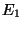=,,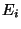=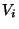,,=. We say that,,,bound a polygon if1.=2.=- 1 ,+ 1 ,In other words, a polygon is a region in the Euclidean plane bounded by line segments without self intersections.However an arbitrary polygon need not be so simple, for instance consider the following polygon: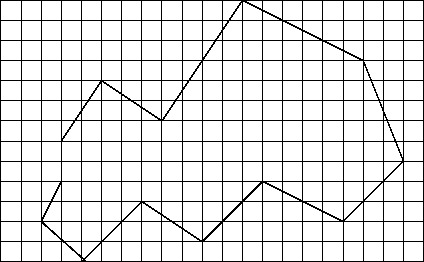How might one go about counting the number of lattice points of a polygon such as the one in figure 2? Well, we can count by hand, which would work for this specific polygon, but suppose we had an even more general polygon than the one in figure two? One way to go about this is to see if we can break the polygon into a less complicated one. Perhaps we can take an arbitrary polygon and partition it into triangles as figure 3 suggests.If we can prove that every simple polygon (a polygon without holes) can be partitioned into triangles, then given a polygon P, the number of lattice points of such a polygon would be the number of lattice points given by each triangle minus the number of lattice points on each diagonal. Indeed, every simple polygon can be triangulated, but to prove this we first need to be versed with more definitions concerning polygons.

Definition: Triangulation

A partitioning of a polygon into triangles s.t. any pair of such triangles intersects on:

1. A common edge
2. A common vertex
3. Empty set

Definition

A vertex on a polygon defines two angles a non-internal angle and an internal angle the angle that intersects the interior of P is called the internal angle.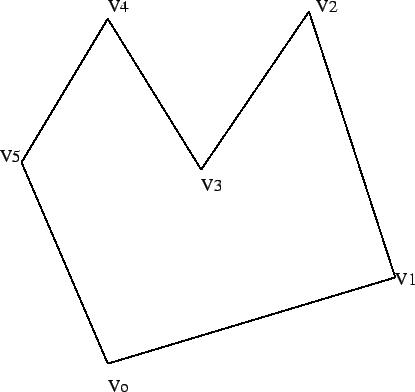Definition: Reflex/Convex Vertex

A vertex V of a polygon is a reflex vertex if its internal angle is strictly greater than. Otherwise the vertex is called convex.Lemma 1

Every polygon must have at least one strictly convex vertex.

Proof

Order the vertices from top to bottom by their correspondingcoordinates. Now, consider the lowest vertices from this list, next pick the right most vertex and suppose that such vertex is reflex. However, if the vertex was reflex then it would contradict the fact that what we have picked the right most vertex. So what we have is indeed a strictly convex vertex. qed.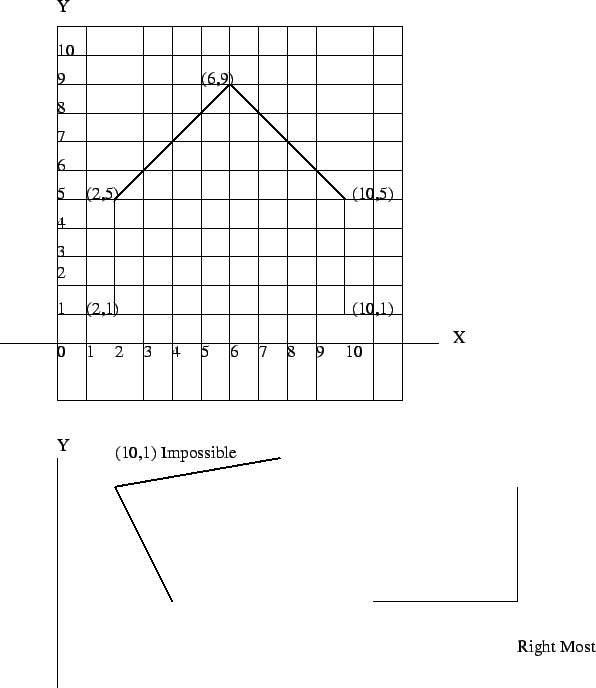Definition: Diagonal

We say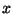can see a pointin a polygon P if the segmentis fully contained in P. A diagonal then, is the line segment joining two vertices that can see each other.

Lemma 2

Every polygon P has at least one diagonal.

Proof

Letbe a strictly convex vertex of a polygon P. The existence of such a vertex was established by lemma 1. Now, let a,b be vertices adjacent to. if a sees b then we are done. Otherwise, vab has at least one vertexin its interior or possibly on its boundary. Takeclosest to. Where the distance is measured perpendicular to the line ab. Suppose now we take a line parallel to ab and move it towards the interior of the polygon so that the last vertex we hit is. Nowmust be a diagonal since there are no points fromto.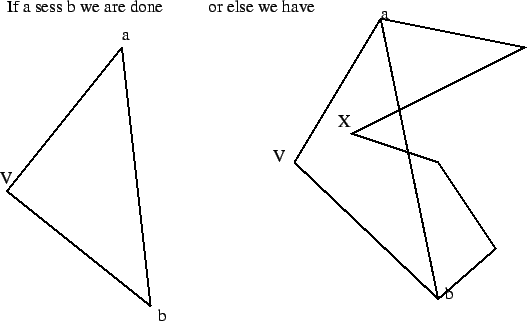we will also need the fact that every polygon must divide the plane. Now finally we get to what will actually help us count the lattice points of an arbitrary polygon P.

theorem 1   Every simple polygon ofvertices maybe partitioned into triangles by the addition of diagonals.

Proof

We will do a proof by induction on the number n4 of sides of a Polygon. Let n = 4 then this is just a square where we can draw a diagonal from one vertex to the opposite vertex and we have our triangulation. Now, suppose. Then such Polygon P will have a diagonal by lemma 2. Pick the one that divides P into two regions. Call the left region polygon A and call the right region polygon B. Polygon B has fewer vertices than the original polygon P. Also Polygon A has fewer vertices than the original Polygon P. Now look at polygon B. Then Draw a diagonal inside B, such diagonal will partition the polygon B into yet another two regions, pick one of them and draw yet another diagonal. Repeat the same process over and over again, since the number of vertices is finite the number of available vertices that we have get smaller and smaller every time we draw a diagonal. Hence, we will eventually reach a point where the polygon B is fully triangulated. Repeat the same process for Polygon A and the original Polygon will be fully triangulated as we wanted.By the triangulation theorem, we have that we can partition a given polygon into triangles. But, how many triangles are there in a given triangulation. And remember that a triangulation will introduce an entire set of diagonals; how many diagonals are there?

Corollary

Every triangulation of a Polygon P ofvertices uses- 3 diagonals and- 2 triangles.

Proof

We will proceed by induction on the number of vertices. Where4. So suppose, then we are talking about a square. Partition this square into triangles now count the number of triangles, there are exactly 4 - 2 = 2 triangles and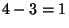diagonal. So for4 we have that such triangulation hasdiagonals andedges. Suppose now4. Then for any triangulation of a Polygon P, Euler's formulawhere V denotes the number of vertices, E denotes the number of edges, and T denotes the number of triangles. Suppose that the claim is true for some4. Then Euler's formula should hold. Thus we can setSo now suppose this is true for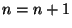. If Euler's formula still holds then we are done. SoSo by P.M.I the conclusion follows. qed.

Now we can set up an algorithm to count the number of lattice points in a given Polygon P.

1. Triangulate P (without adding new vertices)
2. Count the number of lattice points given by each triangle, add them up then store them in a variable say R
3. Count the number of lattice points on each diagonal, add these up and subtract from R
4. Return number
• Mathematics • The University of California, Davis •
• One Shields Avenue • Davis, CA 95616 •